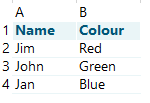Excel is a great tool, but what do you do if you need to find and a return a value in your dataset? That’s where lookup functions come in.

There are a variety of ways to search for, and then return, a value to the cell you’re working in. The simplest of these are:

• VLOOKUP—a function used to lookup values in a column; and
• HLOOKUP—a function used to lookup values in a row.VLOOKUP is a great function and allows you to find a value you’re looking for within one column and, if needed, return a value which is in a neighbouring column. For example, you might be looking up a person in your first column (e.g. John) and then checking to see what their favourite colour is in the next column is (e.g. Green).

The syntax, or how your construct the formula which uses this function, is as follows:

=VLOOKUP(value, table, col_index, [range_lookup])

e.g.       =VLOOKUP(“John”, A2:B4, 2, FALSE)

where John is the name you’re looking up in the first column of your array, A2:B4 is your array, and 2 is the number of the column in the array where the information you want to return is located.

This function only works vertically, hence the V in VLOOKUP. If you’re working within rows, then you’ll want to use HLOOKUP instead.

These are classic Excel functions and have been in the game since 1985. Last year, Excel finally got an upgrade—the introduction of the XLOOKUP function. This new function can lookup both vertically and horizontally and offers some other features to power users.

The syntax for XLOOKUP is: